# AP Physics 1 Practice Test 18

### Test Information10 questions18 minutes

1.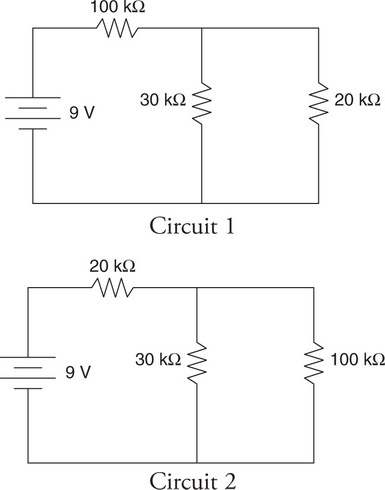The circuits shown in the figure contain the same three resistors connected in different configurations. Which of the following correctly explains which configuration (if either) produces the larger current coming from the battery?

2. A student pushes a puck across a table, moving it from position x = 0 to position x = 0.2 m. After he lets go, the puck continues to travel across the table, coming to rest at position x = 1.2 m. When the puck is at position x = 1.0 m, which of the following is a correct assertion about the net force on the puck?

3.In an experiment, a cart is placed on a flat, negligible-friction track. A light string passes over a nearly ideal pulley. An object with a weight of 2.0 N hangs from the string. The system is released, and the sonic motion detector reads the cart’s acceleration. Can this setup be used to determine the cart’s inertial mass?

4.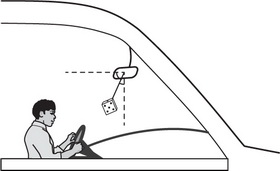An object hangs by a string from a car’s rearview mirror, as shown in the figure. The car is speeding up and moving to the right. Which of the following diagrams correctly represents the forces acting on the object?

5.The diagram shown here represents the particles in a longitudinal standing wave. Which of the following is an approximate measure of the standing wave’s maximum amplitude?

6.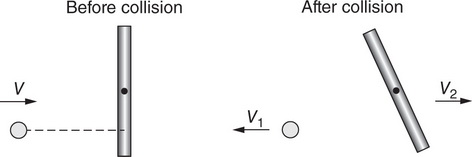Question below refers to the following information:

A small ball of mass m moving to the right at speed v collides with a stationary rod, as shown in the figure. After the collision, the ball rebounds to the left with speed v1, while the rod’s center of mass moves to the left at speed v2. The rod also rotates counterclockwise.

Which of the following equations determines the rod’s change in angular momentum about its center of mass during the collision?

7.Question below refers to the following information:

A small ball of mass m moving to the right at speed v collides with a stationary rod, as shown in the figure. After the collision, the ball rebounds to the left with speed v1, while the rod’s center of mass moves to the left at speed v2. The rod also rotates counterclockwise.

Is angular momentum about the rod’s center of mass conserved in this collision?

8.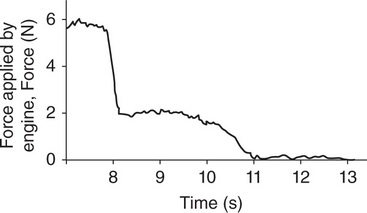Question below refers to the following information:

A model rocket with a mass of 100 g is launched straight up. Eight seconds after launch, when it is moving upward at 110 m/s, the force of the engine drops as shown in the force-time graph.

Which of the following is the best estimate of the impulse applied by the engine to the rocket after the t = 8 s mark?

9.Question below refers to the following information:

A model rocket with a mass of 100 g is launched straight up. Eight seconds after launch, when it is moving upward at 110 m/s, the force of the engine drops as shown in the force-time graph.

Which of the following describes the motion of the rocket between t = 8 s and t = 10 s?

10.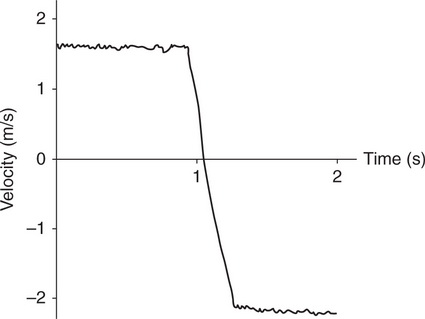The velocity-time graph shown here represents the motion of a 500 g cart that initially moved to the right along a track. It collided with a wall at approximately time (t) = 1.0 s. Which of the following is the best estimate of the impulse experienced by the cart in this collision?